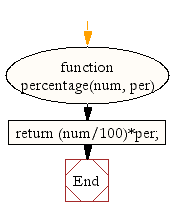# JavaScript: Calculate the percentage of a number

## JavaScript Math: Exercise-32 with Solution

Write a JavaScript function to calculate the percentage (%) of a number.

Test Data:
console.log(percentage(1000, 47.12));
471.2

Sample Solution:-

HTML Code:

``````<!DOCTYPE html>
<html>
<meta charset="utf-8">
<title>JavaScript function to to calculate the percentage (%) of a number</title>
<body>

</body>
</html>
```
```

JavaScript Code:

``````function percentage(num, per)
{
return (num/100)*per;
}

console.log(percentage(1000, 47.12));
```
```

Sample Output:

```471.2
```

Flowchart:Live Demo:

See the Pen javascript-math-exercise-32 by w3resource (@w3resource) on CodePen.

Improve this sample solution and post your code through Disqus

What is the difficulty level of this exercise?

Test your Programming skills with w3resource's quiz.

﻿